Next: 2.3 Carrier Generation and Up: 2. Simulation of Semiconductor Previous: 2.1 Classical Semiconductor Device

Subsections

# 2.2 Analytic MOSFET Approximations

For the simulation of electronic circuits it is often of interest to have compact models for the involved devices. In comparison to solving the semiconductor device equations using compact models reduces the simulation time drastically. Compact models can also be a good guide on what effects the change of physical quantities has on the device characteristics.

The disadvantage is that for each device the right model has to be chosen and the according model parameters have to be extracted. Furthermore, it is not possible to investigate novel device geometries or new materials without processing the device and obtaining an adequate model first.

## 2.2.1 Interface and Oxide Charges

A change in the interface and oxide charges (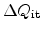and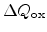) contributes to a threshold voltage shift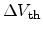as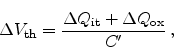(2.31)

where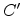is the capacitance per unit area of the oxide.

In the context of NBTI interface charges are usually the result of charged interface defects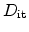. The interface charge depends on the Fermi-level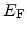and the trap occupancyand can be calculated as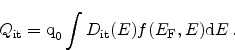(2.32)

Charged oxide traps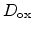contribute to the threshold voltage shift depending on their position in the dielectric. The resulting, effective,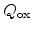can be evaluated as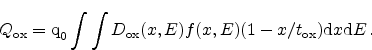(2.33)

Here,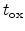is the oxide thickness.

## 2.2.2 The Basic Models

A very common compact model for the MOSFET is the Level 1 model implemented in the circuit simulator SPICE. There the threshold voltage is obtained as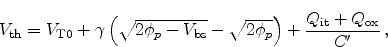(2.34)

where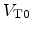is the threshold voltage for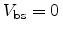V and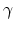the body-effect parameter, defined as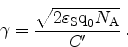(2.35)

Here,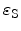is the permittivity of the silicon substrate and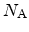the acceptor doping concentration. The potential in the neutral p-type region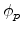is evaluated as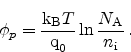(2.36)

During NBTI stress this threshold voltage is shifted due to trapped charges byobtained from (2.31).

In the linear regime, where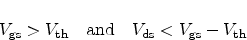(2.37)

the drain current is obtained as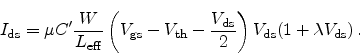(2.38)

Here,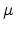is the effective carrier mobility,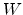the device width,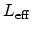the effective gate length, and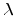is an empirical parameter reflecting the channel length modulation.

In the saturation regime, where(2.39)

the drain current is modeled as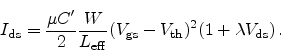(2.40)

## 2.2.3 NBTI Related Models

In NBTI investigations the degradation of the transconductance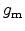is an important figure of merit. The transconductance is defined as the change of drain current as a result of a change in the gate voltage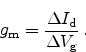(2.41)

Devine et al. proposed a transconductance shift versus interface trap (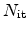), relation as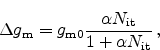(2.42)

where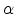is a processing related parameter. As mobility model Devine proposed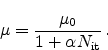(2.43)

With respect to modeling of NBTI these equations show very well how, for example, a reduction of the carrier mobility reduces the drain current, as does an increase of the threshold voltage.Next: 2.3 Carrier Generation and Up: 2. Simulation of Semiconductor Previous: 2.1 Classical Semiconductor Device

R. Entner: Modeling and Simulation of Negative Bias Temperature Instability